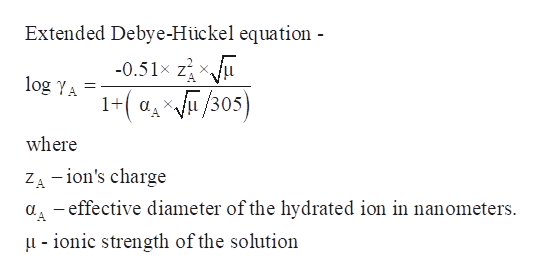# Which statements are true? (Select all that apply.)a) In the ionic strength range 0-0.1 M, activity coefficients increase with decreasing hydrated radius.b)In the ionic strength range 0-0.1 M, activity coefficients decrease with decreasing hydrated radius.c)In the ionic strength range 0-0.1 M, activity coefficients increase with increasing ionic charge.d)In the ionic strength range 0-0.1 M, activity coefficients decrease with increasing ionic charge.d)In the ionic strength range 0-0.1 M activity coefficients increase with increasing ionic strength.e)In the ionic strength range 0-0.1 M activity coefficients decrease with increasing ionic strength.

Question
48 views

Which statements are true? (Select all that apply.)

a) In the ionic strength range 0-0.1 M, activity coefficients increase with decreasing hydrated radius.
b)In the ionic strength range 0-0.1 M, activity coefficients decrease with decreasing hydrated radius.
c)In the ionic strength range 0-0.1 M, activity coefficients increase with increasing ionic charge.d)In the ionic strength range 0-0.1 M, activity coefficients decrease with increasing ionic charge.
d)In the ionic strength range 0-0.1 M activity coefficients increase with increasing ionic strength.
e)In the ionic strength range 0-0.1 M activity coefficients decrease with increasing ionic strength.
check_circle

Step 1

The true statements are to be determined-

1. a) In the ionic strength range 0-0.1 M, activity coefficients increase with decreasing hydrated radius.
2. b) In the ionic strength range 0-0.1 M, activity coefficients decrease with decreasing hydrated radius.
3. c) In the ionic strength range 0-0.1 M, activity coefficients increase with increasing ionic charge.
4. d) In the ionic strength range 0-0.1 M, activity coefficients decrease with increasing ionic charge.
5. e) In the ionic strength range 0-0.1 Mactivity coefficients increase with increasing ionic strength.
6. f) In the ionic strength range 0-0.1 Mactivity coefficients decrease with increasing ionic strength.
Step 2

Ionic strength is defined as the total ion concentration in the solution.

Activity coefficient is a measure of deviation of solution from the ideal behaviour. And it is calculated using the extended Debye-Hückel equation.help_outlineImage TranscriptioncloseExtended Debye-Hückel equation - 0.51x z X. A log YA 1-(a/305) where ZA-ion's charge aeffective diameter of the hydrated ion in nanometers u -ionic strength of the solution fullscreen
Step 3

Hydrated radius is the radius of the ion plus the water molecules that are bounded to the ion.

### Want to see the full answer?

See Solution

#### Want to see this answer and more?

Solutions are written by subject experts who are available 24/7. Questions are typically answered within 1 hour.*

See Solution
*Response times may vary by subject and question.
Tagged in

### Chemistry